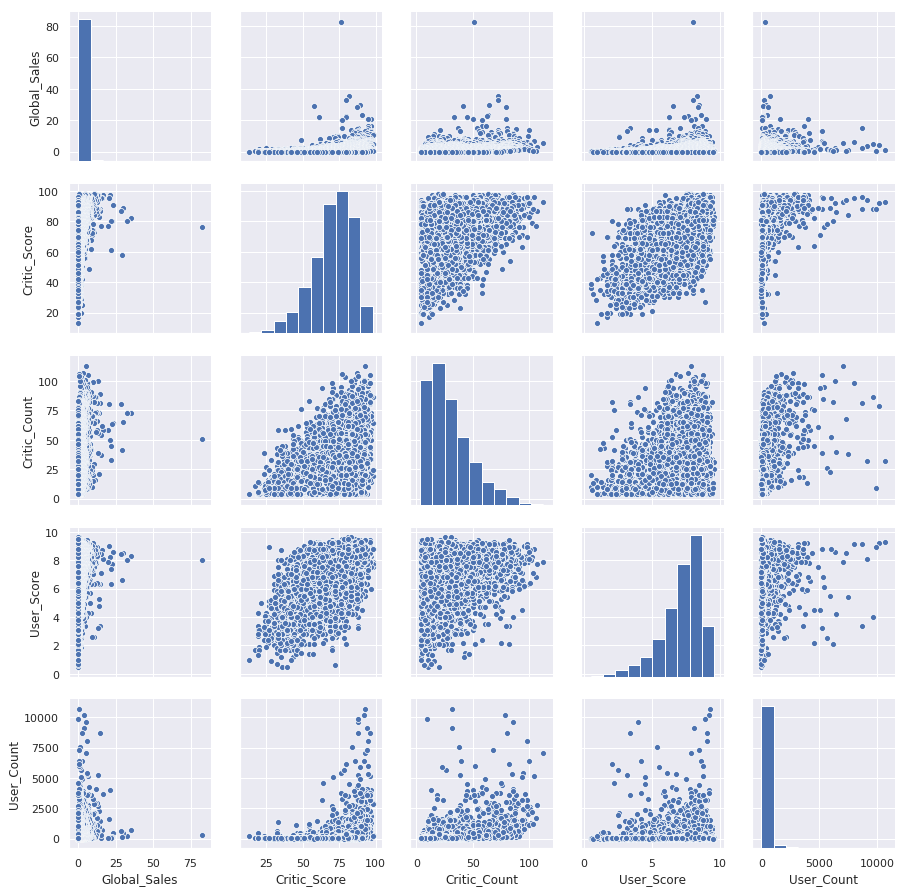## mlcourse.ai – Open Machine Learning Course¶

Author: Egor Polusmak. Translated and edited by Alena Sharlo, Yury Kashnitsky, Artem Trunov, Anastasia Manokhina, and Yuanyuan Pao. This material is subject to the terms and conditions of the Creative Commons CC BY-NC-SA 4.0 license. Free use is permitted for any non-commercial purpose.

# Topic 2. Visual data analysis in Python

## 1. Dataset¶

First, we will set up our environment by importing all necessary libraries. We will also change the display settings to better show plots.

In :
# Matplotlib forms basis for visualization in Python
import matplotlib.pyplot as plt

# We will use the Seaborn library
import seaborn as sns
sns.set()

# Graphics in SVG format are more sharp and legible
%config InlineBackend.figure_format = 'svg'

# Increase the default plot size and set the color scheme
plt.rcParams['figure.figsize'] = (8, 5)
plt.rcParams['image.cmap'] = 'viridis'
import pandas as pd


Now, let’s load the dataset that we will be using into a DataFrame. I have picked a dataset on video game sales and ratings from Kaggle Datasets. Some of the games in this dataset lack ratings; so, let’s filter for only those examples that have all of their values present.

In :
df = pd.read_csv('../../data/video_games_sales.csv').dropna()
print(df.shape)

(6825, 16)


Next, print the summary of the DataFrame to check data types and to verify everything is non-null.

In :
df.info()

<class 'pandas.core.frame.DataFrame'>
Int64Index: 6825 entries, 0 to 16706
Data columns (total 16 columns):
Name               6825 non-null object
Platform           6825 non-null object
Year_of_Release    6825 non-null float64
Genre              6825 non-null object
Publisher          6825 non-null object
NA_Sales           6825 non-null float64
EU_Sales           6825 non-null float64
JP_Sales           6825 non-null float64
Other_Sales        6825 non-null float64
Global_Sales       6825 non-null float64
Critic_Score       6825 non-null float64
Critic_Count       6825 non-null float64
User_Score         6825 non-null object
User_Count         6825 non-null float64
Developer          6825 non-null object
Rating             6825 non-null object
dtypes: float64(9), object(7)
memory usage: 906.4+ KB


We see that pandas has loaded some of the numerical features as object type. We will explicitly convert those columns into float and int.

In :
df['User_Score'] = df['User_Score'].astype('float64')
df['Year_of_Release'] = df['Year_of_Release'].astype('int64')
df['User_Count'] = df['User_Count'].astype('int64')
df['Critic_Count'] = df['Critic_Count'].astype('int64')


The resulting DataFrame contains 6825 examples and 16 columns. Let’s look at the first few entries with the head() method to check that everything has been parsed correctly. To make it more convenient, I have listed only the variables that we will use in this notebook.

In :
useful_cols = ['Name', 'Platform', 'Year_of_Release', 'Genre',
'Global_Sales', 'Critic_Score', 'Critic_Count',
'User_Score', 'User_Count', 'Rating'
]

Out:
Name Platform Year_of_Release Genre Global_Sales Critic_Score Critic_Count User_Score User_Count Rating
0 Wii Sports Wii 2006 Sports 82.53 76.0 51 8.0 322 E
2 Mario Kart Wii Wii 2008 Racing 35.52 82.0 73 8.3 709 E
3 Wii Sports Resort Wii 2009 Sports 32.77 80.0 73 8.0 192 E
6 New Super Mario Bros. DS 2006 Platform 29.80 89.0 65 8.5 431 E
7 Wii Play Wii 2006 Misc 28.92 58.0 41 6.6 129 E

## 2. DataFrame.plot()¶

Before we turn to Seaborn and Plotly, let’s discuss the simplest and often most convenient way to visualize data from a DataFrame: using its own plot() method.

As an example, we will create a plot of video game sales by country and year. First, let’s keep only the columns we need. Then, we will calculate the total sales by year and call the plot() method on the resulting DataFrame.

In :
df[[x for x in df.columns if 'Sales' in x] +
['Year_of_Release']].groupby('Year_of_Release').sum().plot();


Note that the implementation of the plot() method in pandas is based on matplotlib.

Using the kind parameter, you can change the type of the plot to, for example, a bar chart. matplotlib is generally quite flexible for customizing plots. You can change almost everything in the chart, but you may need to dig into the documentation to find the corresponding parameters. For example, the parameter rot is responsible for the rotation angle of ticks on the x-axis (for vertical plots):

In :
df[[x for x in df.columns if 'Sales' in x] +
['Year_of_Release']].groupby('Year_of_Release').sum().plot(kind='bar', rot=45);


## 3. Seaborn¶

Now, let's move on to the Seaborn library. seaborn is essentially a higher-level API based on the matplotlib library. Among other things, it differs from the latter in that it contains more adequate default settings for plotting. By adding import seaborn as sns; sns.set() in your code, the images of your plots will become much nicer. Also, this library contains a set of complex tools for visualization that would otherwise (i.e. when using bare matplotlib) require quite a large amount of code.

#### pairplot()¶

Let's take a look at the first of such complex plots, a pairwise relationships plot, which creates a matrix of scatter plots by default. This kind of plot helps us visualize the relationship between different variables in a single output.

In :
# pairplot() may become very slow with the SVG format
%config InlineBackend.figure_format = 'png'
sns.pairplot(df[['Global_Sales', 'Critic_Score', 'Critic_Count',
'User_Score', 'User_Count']]);As you can see, the distribution histograms lie on the diagonal of the matrix. The remaining charts are scatter plots for the corresponding pairs of features.

#### distplot()¶

It is also possible to plot a distribution of observations with seaborn's distplot(). For example, let's look at the distribution of critics' ratings: Critic_Score. By default, the plot displays a histogram and the kernel density estimate.

In :
%config InlineBackend.figure_format = 'svg'
sns.distplot(df['Critic_Score']);


#### jointplot()¶

To look more closely at the relationship between two numerical variables, you can use joint plot, which is a cross between a scatter plot and histogram. Let's see how the Critic_Score and User_Score features are related.

In :
sns.jointplot(x='Critic_Score', y='User_Score',
data=df, kind='scatter');